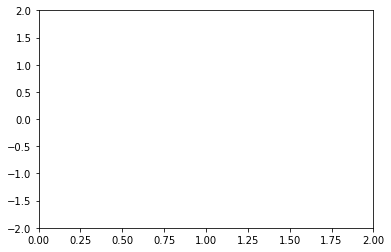# Embedding Matplotlib Animations in Jupyter as Interactive JavaScript Widgets

A while back I wrote a post on Embedding Matplotlib Animations in Jupyter Noteboks, which became surprisingly popular. It outlined how to render Matplotlib animations in the Jupyter Notebook, by encoding it as a HTML5 video using the to_html5_video method introduced in the release of Matplotlib 1.5.

Three years have gone by since then, and much has changed in the world of open-source scientific Python. Matplotlib 2.1 was released towards the end of last year, and was the first release with major new features since 1.5. Among many significant enhancements, the release included the merge of Jake Vanderplas' JSAnimation package, a tool for rendering Matplotlib animations as interactive JavaScript widgets.

With this awesome addition, encapsulated in the new to_jshtml method, we are now able to embed an interactive JavaScript widget for playback of Matplotlib animations directly inside a Jupyter Notebook.

In this post, we demonstrate how to use this method on the same example animation from my previous post, which was originally derived from an even earlier post by Jake VanderPlas.

In :
%matplotlib inline

In :
import numpy as np
import matplotlib.pyplot as plt

from matplotlib import animation, rc
from IPython.display import HTML


### Creating the Animation¶

First set up the figure, the axis, and the plot element we want to animate:

In :
fig, ax = plt.subplots()

ax.set_xlim(( 0, 2))
ax.set_ylim((-2, 2))

line, = ax.plot([], [], lw=2)Define the initialization function, which plots the background of each frame:

In :
def init():
line.set_data([], [])
return (line,)


Define the animation function, which is called for each new frame:

In :
def animate(i):
x = np.linspace(0, 2, 1000)
y = np.sin(2 * np.pi * (x - 0.01 * i))
line.set_data(x, y)
return (line,)


Compile the animation. Setting blit=True will only re-draw the parts that have changed.

In :
anim = animation.FuncAnimation(fig, animate, init_func=init,
frames=100, interval=20,
blit=True)


### Displaying the Animation¶

Now we can display this anim object using IPython's HTML display method. For comparison, we first encode the animation and render it as an HTML5 video using the to_html5_video method, as covered in my previous post:

In :
HTML(anim.to_html5_video())

Out:

Now to create an interactive JavaScript widget using the to_jshtml method:

In :
HTML(anim.to_jshtml())

Out:

Once Loop Reflect